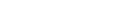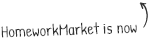•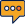0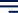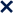# Mathematics homework helpGet help from top-rated tutors in any subject.

Math has always been a tough subject to study. Keep in mind that mathematics is the core of many scientific studies, such as the study of our universe. In essence, math is as important as any other subject you may need to study.

However, we understand that math is not for everyone. In fact, many students struggle with mathematics homework each day; that's why we're here to help.

There's no shame in asking for math homework help. When it comes to mathematics, there are endless possibilities to go with, making it a dense subject for some people. However, we've worked with several math areas over the years, and we're positive we can help you with your homework.

Our specialized math tutors are here to walk you through every step of the process. Typically, we get requests for the following math areas:

• Algebra
• Geometry
• Statistics
• Calculus
• Trigonometry
• Probability

While those are the most common math assignments we get, there are several other areas that we can help you with. If you want to know how we can help you, simply talk to one of our tutors, and let's get started!

## Why Would You Benefit from Getting Homework Help from a Tutor?

There are many reasons why you would need math homework help. In most cases, people request help because they're confused about some technical terms, or they don't have enough time to finish their homework.

Regardless of the reason you're struggling with your homework, we can help you. To work with us, choose the tutor of your preference and get to work; it's that easy! We guarantee high-quality results in record time. Time is one of the most important things you have in life, so saving as much as possible can make all the difference.

If you're eager to get help with your math homework, try contacting one of our experts and let us help you!